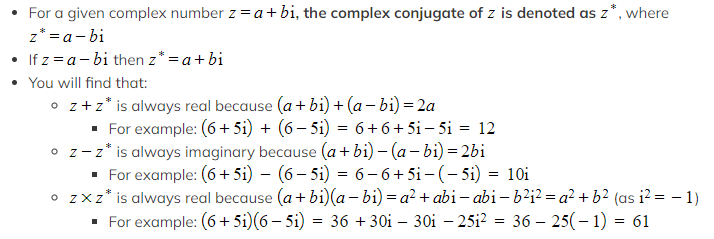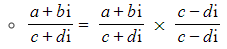# CIE A Level Maths: Pure 3复习笔记8.1.2 Complex Conjugation & Division

### Complex Conjugation & Division

When dividing complex numbers, we can use the complex conjugate to make the denominator a real number, which makes carrying out the division much easier.

####How do I divide complex numbers?

• When we divide complex numbers, we can express the calculation in the form of a fraction, and then start by multiplying the top and bottom by the conjugate of the denominator:
•This ensures we are multiplying by 1; so not affecting the overall value
• This gives us a real number as the denominator because we have a complex number multiplied by its conjugate ([Image_Link]data:;base64,